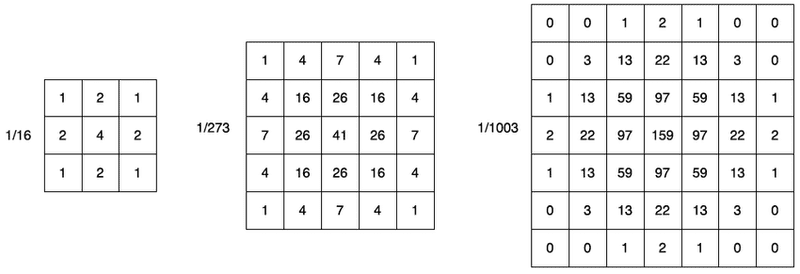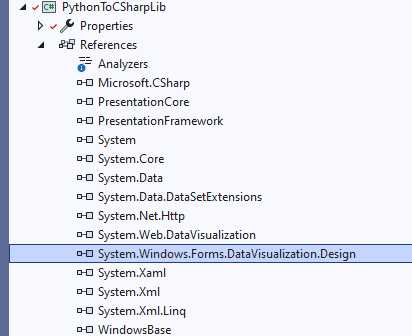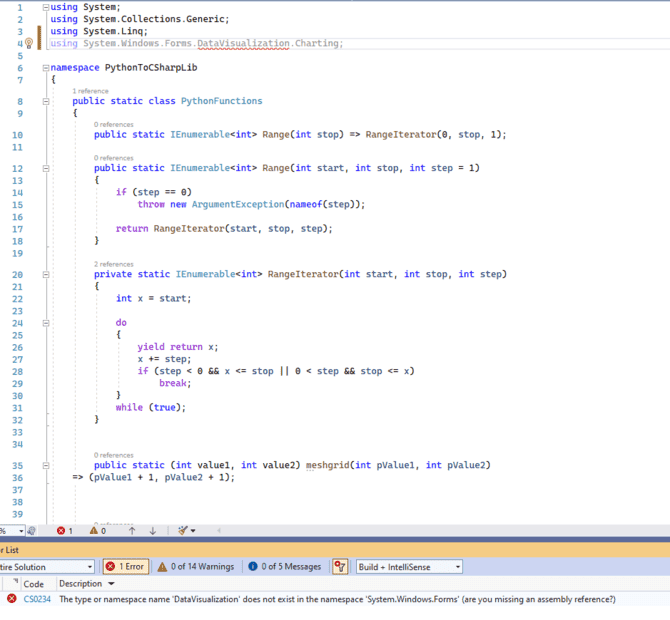# How close to Gaussian a 2D Matrix percentage is in C#

• C#
• btb4198

#### btb4198

Does anyone know a C# class that can return a value (0 - 100 percentage) of How close a perfect gaussian curve an 2D Matrix is?

for example, these would all return a 100%:What do the percentages mean? Are they related to probabilities? or confidence numbers?
I can only think of the Chi-square goodness of fit method to measure how close a sample histogram is to a theoretical distribution. But that gives a number that allows you to conclude that a certain sample would be unlikely to fit a distribution that represents the null hypothesis. Using it to PROVE that the sample IS from the given distribution is misusing the statistic. It only allows you to continue to SUPPOSE that it is from the given distribution.

What do the percentages mean? Are they related to probabilities? or confidence numbers?
I can only think of the Chi-square goodness of fit method to measure how close a sample histogram is to a theoretical distribution. But that gives a number that allows you to conclude that a certain sample would be unlikely to fit a distribution that represents the null hypothesis. Using it to PROVE that the sample IS from the given distribution is misusing the statistic. It only allows you to continue to SUPPOSE that it is from the given distribution.
I am doing grading( 100% -0) 100-90 is a A, 89- 80 is a B, 79-70 C, and 60 on down is a F

What do the percentages mean? Are they related to probabilities? or confidence numbers?
I can only think of the Chi-square goodness of fit method to measure how close a sample histogram is to a theoretical distribution. But that gives a number that allows you to conclude that a certain sample would be unlikely to fit a distribution that represents the null hypothesis. Using it to PROVE that the sample IS from the given distribution is misusing the statistic. It only allows you to continue to SUPPOSE that it is from the given distribution.

They have this :

> First, let's create a 2D matrix with some random data. We'll use the
> System.Random class to generate pseudo-random numbers:

C++:
var rand = new Random();
var matrix = new double[5,5];
for(int i=0; i < matrix.GetLength(0); i++)
{
for(int j=0; j < matrix.GetLength(1); j++)
{
matrix = rand.NextDouble() * 100;
}
}

> Now that we have our data, we can calculate the mean and standard
> deviation:

C++:
double mean = matrix.Average();
double stdDev = Math.Sqrt(matrix.Variance());

but when I tried that in C# does, I get this error:

Severity Code Description Project File Line Suppression State
Error CS1061 'double[*,*]' does not contain a definition for 'Variance' and no
accessible extension method 'Variance' accepting a first argument of type 'double[*,*]'
could be found (are you missing a using directive or an assembly reference?)

using System.Numerics;

but it did not help

Last edited by a moderator:
I do not have a C# compiler and I can not find any evidence (using Google) that C# has a Variance method for an array. All I see are people defining their own variance or standard deviation program as in this link.

• What are the two independent variables (i.e. what do the rows and columns represent - exam marks are usually modeled by a Gaussian with a 1D domain).
• What is the dependent variable (i.e. what do the values in the cells represent)?
• When you use code tags, please add lang=cpp to the opening tag (or select C in the menu if you are using the wysiwyg editor button) so it highlights c-like syntax.
What article?

I do not have a C# compiler and I can not find any evidence (using Google) that C# has a Variance method for an array. All I see are people defining their own variance or standard deviation program as in this link.
I found this StatisticFormula
but when I add it to my WPF project, I get an error: it can't find it, but added the .dll.
So I Tried using System.Web.UI.DataVisualization.Charting;
but it found that, but it still can't find Variance.

I found this StatisticFormula
but when I add it to my WPF project, I get an error: it can't find it, but added the .dll.
So I Tried using System.Web.UI.DataVisualization.Charting;
but it found that, but it still can't find Variance.
As you can see from the name, members of that namespace are used for plotting data in a web app: is that what you are doing? How much experience do you have with C#?

As you can see from the name, members of that namespace are used for plotting data in a web app: is that what you are doing? How much experience do you have with C#?
if you look at the original link, is says :
Code:
Namespace:
System.Windows.Forms.DataVisualization.Charting
Assembly:
System.Windows.Forms.DataVisualization.dll

I added that .dll and I tried that using but it says:
Code:
Severity    Code    Description    Project    File    Line    Suppression State
Error    CS0234    The type or namespace name 'DataVisualization' does not exist in the namespace 'System.Windows.Forms' (are you missing an assembly reference?)

So I was looking for a WPF one but I only find that Web one and I was just trying it.

So I Tried using System.Web.UI.DataVisualization.Charting;
but it found that, but it still can't find Variance.

if you look at the original link, is says :
Code:
Namespace: System.Windows.Forms.DataVisualization.Charting
Assembly: System.Windows.Forms.DataVisualization.dll
Right, but it doesn't say System.Web.UI.DataVisualization.Charting, which is what you said you tried.
It seems to me that if you include the correct namespace, Visual Studio will link in the appropriate DLL. I don't think you need to do this yourself.

btb4198 said:
I added that .dll and I tried that using but it says:
Code:
Severity    Code    Description    Project    File    Line    Suppression State
Error    CS0234    The type or namespace name 'DataVisualization' does not exist in the namespace 'System.Windows.Forms' (are you missing an assembly reference?)

So I was looking for a WPF one but I only find that Web one and I was just trying it.
Did you specify the full namespace? I.e.,
System.Windows.Forms.DataVisualization.Charting?

Are you sure you know what you are doing here, both from a statistical and a coding point of view? Can you answer the background questions @FactChecker and I have answered, and provide a link to the article you are trying to follow?

Are you sure you know what you are doing here, both from a statistical and a coding point of view? Can you answer the background questions @FactChecker and I have answered, and provide a link to the article you are trying to follow?
I posted the question here and got the right answer which out anyone questions my background. They just answered the question, it was really nice and easy. You can close this post.
Thanks

Right, but it doesn't say System.Web.UI.DataVisualization.Charting, which is what you said you tried.
It seems to me that if you include the correct namespace, Visual Studio will link in the appropriate DLL. I don't think you need to do this yourself.

Did you specify the full namespace? I.e.,
System.Windows.Forms.DataVisualization.Charting?
Mark44,
Yes I did. but it did not work.
see:andI added both the .dll and using.
I do not know why it cannot find it.
It really sucks because that lib would be very very useful to me.

You can close this post.
Done.

Mark44 said:
Did you specify the full namespace? I.e.,
System.Windows.Forms.DataVisualization.Charting?
Mark44,
Yes I did. but it did not work.
@btb198, one possibility is that in the tutorial you were reading, they were using a different version of the .NET framework than the one you have on your computer. That's the only reason I can think of as to why Visual Studio was unable to find the DataVisualization class (namespace?).